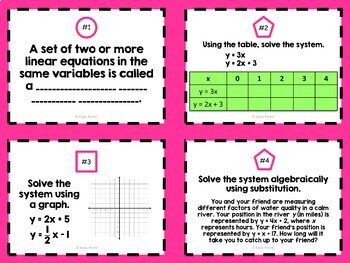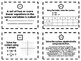# Solving Systems of Equations Task CardsSubject
Resource Type
File Type

Zip

(810 KB|10 pages)
Product Rating
Standards
• Product Description
• StandardsNEW

These solving systems of linear equations task cards provide an engaging review that will allow students to get out of their seats and have fun with their learning. These task cards have a focus on key vocabulary and a variety of problems that help students practice their skills with this topic. These task cards cover common core standard 8.EE.8.

BEFORE YOU BUY: Be sure to look at the growing 8th grade math task card bundle to see if this is a better option for you.

Task Cards will cover the following:

• understanding that the solution of two linear equations corresponds with the intersection
• solving systems of two linear equations in two variables algebraically
• solving real-world and mathematical problems leading in two variables
• solving systems of linear equations by elimination and substitution
• solving systems of linear equations by graphing and by a table
• one solution, no solution, infinitely many solutions
• and more!

The task cards in this set are categorized by difficulty level.

• A CIRCLE is the easiest level.
• A SQUARE is an intermediate level.
• A PENTAGON is the hardest level.

You can choose to have all students complete each task card OR divide the task cards up for different groups of students. The possibilities are endless!

There are also interdisciplinary aspects in these task cards, including science and writing!!

These task cards are great for RTI, Common Core Review, Regular Class Review, Test Prep, Engagement, “I’m Finished” stations, Math Centers, and More!

There are 24 task cards in all. An answer sheet and answer key is included. A black and white copy is included as well.

★ Check out my other related products!

Solving Sytems of Linear Equations: Substitution Cut-and-Glue Activity

✦✦✦✦✦✦✦✦✦✦✦✦✦✦✦✦✦✦✦✦✦✦✦✦✦✦✦✦✦✦✦✦✦✦✦✦✦✦✦✦✦✦✦✦✦✦✦✦✦

Be sure to follow my store for updates on new products and sales.

Also, don't forget to leave feedback. You will receive TPT credits that can be used for future purchases!

Solve real-world and mathematical problems leading to two linear equations in two variables. For example, given coordinates for two pairs of points, determine whether the line through the first pair of points intersects the line through the second pair.
Solve systems of two linear equations in two variables algebraically, and estimate solutions by graphing the equations. Solve simple cases by inspection. For example, 3𝘹 + 2𝘺 = 5 and 3𝘹 + 2𝘺 = 6 have no solution because 3𝘹 + 2𝘺 cannot simultaneously be 5 and 6.
Understand that solutions to a system of two linear equations in two variables correspond to points of intersection of their graphs, because points of intersection satisfy both equations simultaneously.
Analyze and solve pairs of simultaneous linear equations.
Total Pages
10 pages
Included
Teaching Duration
N/A
Report this Resource to TpT
Reported resources will be reviewed by our team. Report this resource to let us know if this resource violates TpT’s content guidelines.